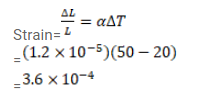# A steel rod is clamped at its two ends and rests on fixed horizontal base.Question:

A steel rod is clamped at its two ends and rests on fixed horizontal base. The rod is unstrained at $20^{\circ} \mathrm{C}$ Find the longitudinal strain developed in the rod if the temperature rises to $50^{\circ} \mathrm{C}$. Coefficient of linear expansion of steel $=1.2^{\times 10^{-5} /{ }^{\circ} \mathrm{C}}$

Solution: